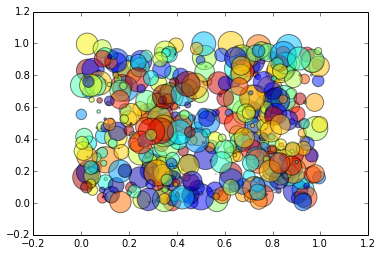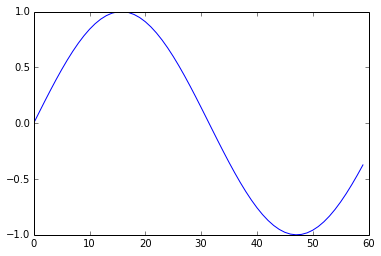CoCalc Public Filessupport / 2014-12-21-ipython.html
Authors: Harald Schilly, ℏal Snyder, William A. Stein
Description: Jupyter notebook support/2015-06-04-141749-bokeh.ipynb
2014-12-21-ipython

This is a simple example of an IPython notebook, which I Published using SageMathCloud's new "Publish" button.

In :
import numpy as np
import matplotlib.pyplot as plt
N = 500
x = np.random.rand(N); y = np.random.rand(N)
colors = np.random.rand(N)
area = np.pi * (15 * np.random.rand(N))**2 # 0 to 15 point radiuses
plt.scatter(x, y, s=area, c=colors, alpha=0.5)
plt.show()In :
import math
plot([math.sin(x/10.) for x in range(0,60)])

Out:
[<matplotlib.lines.Line2D at 0x7f0312203810>]In []: